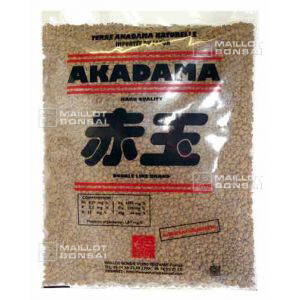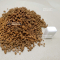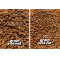##### The Japanese Bonsai specialist
Direct order Contact Help / Services Newsletter# Akadama bonsai soil 2ltr bag normal grain

› Bonsai soil and fertiliser › Bonsai soilref. : 5271

6,10

voluminous/heavy item extra shipping of 3,00

Available quantity : 22Order

###### Description

Multi-purpose normal grain bonsai soil used to grow many species.

Grain size Ø + -2/3mm and 4mm. Small sachet + - 2 litres. SUPERIOR QUALITY, DRIED FOR MORE THAN 2 YEARS. Weight according to the rate of moisture of +- 1.5 kilos

Origin: natural volcanic earth from the mountainous regions of Japan.

Benefits: soil drainage and root ventilation for the healthy growth of your bonsai.

How to use:use alone, with Kanuma earth for acers, or add gravel and large-grained sand but not compost. This earth is not very nourishing and a Biogold fertiliser should be used regularly. You'll need to water this large-grained soil more in summer.

Species: for all conifer and caduc trees (for rhododendrons and azaleas you'll need Kanuma earth).

#soil 7.4 #bonsai 6.6 #grain 4.5 #earth 4.5 #normal 3.6 #species 2.7 #akadama 2.7 #grained 2.7 #kanuma 2.6 #large 2.5

Formule
(( ROUND((CHAR_LENGTH(b.article_nom)-CHAR_LENGTH(REPLACE(b.article_nom, 'soil', '')))/LENGTH('soil')) + ROUND((CHAR_LENGTH(b.article_description)-CHAR_LENGTH(REPLACE(b.article_description, 'soil', '')))/LENGTH('soil')) ) * 5.4) + (( ROUND((CHAR_LENGTH(b.article_nom)-CHAR_LENGTH(REPLACE(b.article_nom, 'bonsai', '')))/LENGTH('bonsai')) + ROUND((CHAR_LENGTH(b.article_description)-CHAR_LENGTH(REPLACE(b.article_description, 'bonsai', '')))/LENGTH('bonsai')) ) * 4.6) + (( ROUND((CHAR_LENGTH(b.article_nom)-CHAR_LENGTH(REPLACE(b.article_nom, 'grain', '')))/LENGTH('grain')) + ROUND((CHAR_LENGTH(b.article_description)-CHAR_LENGTH(REPLACE(b.article_description, 'grain', '')))/LENGTH('grain')) ) * 4.5) + (( ROUND((CHAR_LENGTH(b.article_nom)-CHAR_LENGTH(REPLACE(b.article_nom, 'earth', '')))/LENGTH('earth')) + ROUND((CHAR_LENGTH(b.article_description)-CHAR_LENGTH(REPLACE(b.article_description, 'earth', '')))/LENGTH('earth')) ) * 4.5) + (( ROUND((CHAR_LENGTH(b.article_nom)-CHAR_LENGTH(REPLACE(b.article_nom, 'normal', '')))/LENGTH('normal')) + ROUND((CHAR_LENGTH(b.article_description)-CHAR_LENGTH(REPLACE(b.article_description, 'normal', '')))/LENGTH('normal')) ) * 3.6) + (( ROUND((CHAR_LENGTH(b.article_nom)-CHAR_LENGTH(REPLACE(b.article_nom, 'species', '')))/LENGTH('species')) + ROUND((CHAR_LENGTH(b.article_description)-CHAR_LENGTH(REPLACE(b.article_description, 'species', '')))/LENGTH('species')) ) * 2.7) + (( ROUND((CHAR_LENGTH(b.article_nom)-CHAR_LENGTH(REPLACE(b.article_nom, 'akadama', '')))/LENGTH('akadama')) + ROUND((CHAR_LENGTH(b.article_description)-CHAR_LENGTH(REPLACE(b.article_description, 'akadama', '')))/LENGTH('akadama')) ) * 2.7) + (( ROUND((CHAR_LENGTH(b.article_nom)-CHAR_LENGTH(REPLACE(b.article_nom, 'grained', '')))/LENGTH('grained')) + ROUND((CHAR_LENGTH(b.article_description)-CHAR_LENGTH(REPLACE(b.article_description, 'grained', '')))/LENGTH('grained')) ) * 2.7) + (( ROUND((CHAR_LENGTH(b.article_nom)-CHAR_LENGTH(REPLACE(b.article_nom, 'kanuma', '')))/LENGTH('kanuma')) + ROUND((CHAR_LENGTH(b.article_description)-CHAR_LENGTH(REPLACE(b.article_description, 'kanuma', '')))/LENGTH('kanuma')) ) * 2.6) + (( ROUND((CHAR_LENGTH(b.article_nom)-CHAR_LENGTH(REPLACE(b.article_nom, 'large', '')))/LENGTH('large')) + ROUND((CHAR_LENGTH(b.article_description)-CHAR_LENGTH(REPLACE(b.article_description, 'large', '')))/LENGTH('large')) ) * 2.5)

## Secure payment## Delivery

Our logistic partners :04 74 55 23 48
Pépinière MAILLOT-BONSAÏ
Le Bois Frazy
01990 RELEVANT - FRANCE
on appointment